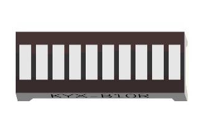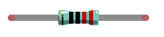# Knight Rider pattern with 10 LED bar graph Display

## In this tutorial, I'll show you how to control a simple 10 LED Bar Graph display with Arduino. This type of bar graph is basically just 10 LEDs in a row with anodes on one side of the component and cathodes on the othersLet us use Arduino UNO to control a bar graph LED consist of 10 LEDs.

### Component listArduino UNO x1 Buy NowBreadboard x1 Buy NowUSB cable x1 Buy NowLED bar Graph Display Buy NowResistor 220Ω x2 Buy NowJumper M/M x3 Buy Now

## Component knowledge

Let us learn about the basic features of components to use them better.

### LED bar graph

LED bar graph is a component Integration consist of 10 LEDs. There are two rows of pins at its bottom. At the bottom of the LED bar graph, there are two rows of pins, corresponding to the positive and negative pole separately. If the LED bar graph can not work in the circuit, it was probably because the connection between positive and negative pole is wrong. Please try to reverse the LED bar graph connection.## Code knowledge

This section will use new code knowledge.

### Array

The array is used to record a set of variables. An array is defined below:

``int a;  ``

"int" is the type of the array and "10" is an element of the array. This array can store 10 int types of elements as below.

``int a = {0, 1, 2, 3, 4, 5, 6, 7, 8, 9}; ``

Or there is another form that the number of elements is the size of the array:

``int a[] = {0, 1, 2, 3, 4, 5, 6, 7, 8, 9}; ``

We can reference elements of an array as below：

``````int i, j;
i = a;
j = a;
a = 0;``````

Among them, "[]" is the array index, with a as the first elements in the array.

For example, now we define an array b[] below:

``int b[] = {5, 6, 7, 8,4,7,9,1,2,0};``

The value of each element in array b[] is as follows:b b b b 5 6 7 8

This is just the use of a one-dimensional array. And there are two-dimensional arrays, three-dimensional arrays, and multi-dimensional arrays. Readers interested of this part can develop your own learning.

### Loop

The loop statement is used to perform repetitive work such as the initialization to all the elements of an array.

``````while(expression)
functionX();``````

When there is more than one statement to be executed, the form is as follows:

``````while(expression){
functionX();
functionY();
}``````

The first step of the execution is judgment expression inside "()". If the result is false, the statements inside "{}"

will not be executed; if the result is true, the statements will be executed.

``````int i = 0;
while (i < 2)
i = i + 1;
i = 5;``````
• First time: i<2, i=0 is tenable, execute i=i+1, then i=1;
• Second time: i<2, i=1 is tenable, execute i=i+1, then i=2;
• Third time: i<2, i=2 is not tenable, execution of loop statements is completed. Statement i=5 will be executed next.

"do while" and "while" is similar. The difference is that the loop statements of "do while" is executed before judging expression. The result of the judgment will decide whether or not go on the next execution:

``````do {
functionX();
} while (expression);``````

"for" is another loop statement, and its form is as follows:

``````for (expression1; expression2; expression 3)
functionX();``````

When there is more than one statement to be executed, the form is as follows:

``````for (expression 1; expression 2; expression 3) {
functionX();
functionY();
}``````

Expression 1 is generally used to initialize variables; expression 2 is the judgment which is used to decide whether or not to execute loop statements; the expression 3 is generally used to change the value of variables.

For example:

``````int i = 0, j = 0;
for (i = 0; i < 2; i++)
j++;
i = 5;``````
• First: i=0, i<2 is tenable, execute j++, and execute i++, then i=1, j=1;
• Second: i=1, i<2 is tenable, execute j++, and execute i++, then i=2, j=2;
• Third times: i<2 is tenable, i=2 is not tenable. The execution of loop statements is completed. Statement i=5 will be executed next.
 Operator ++, -- "i++" is equivalent to "i=i+1". And "i--" equivalent to "i=i-1".

## Circuit

Let us use D3, D4, D5, D6, D7, D8, D9, D10, D11, D12 pin of Arduino UNO board to drive LED bar graph.Hardware connection

## Sketch

Now let us complete the sketch to control the LED bar graph.

#### Sketch 1

First, write a sketch which can achieve the LED light water

``````const int ledCount = 10;    // the number of LEDs in the bar graph

// an array of pin numbers to which LEDs are attached
int ledPins[] = { 3, 4, 5, 6, 7, 8, 9, 10, 11, 12 };

void setup() {
// loop over the pin array and set them all to output:
for (int i = 0; i < ledCount; i++) {
pinMode(ledPins[i], OUTPUT);
}
}

void loop() {
// the ith LED of LED bar graph will light up in turn
for (int i = 0; i < ledCount; i++) {
barGraphDisplay(i);
}
}

void barGraphDisplay(int ledOn) {
// make the "ledOn"th LED of bar graph LED on and the others off
for (int i = 0; i < ledCount; i++) {
if (i == ledOn)
digitalWrite(ledPins[i], HIGH);
else
digitalWrite(ledPins[i], LOW);
}
delay(100);
} ``````

Firstly, let us define a read-only variable to record the number of LEDs as the number of times in the loop.

``const int ledCount = 10; // the number of LEDs in the bar graph``
 Read-only variable "const" keyword is used to define read-only variables, which is called in the same way with comen variable. But read-only variables can be assigned only once.

Then we define an array used to store the number of pins connected to LED bar graph. So it is convenient to manipulate arrays to modify the pin number.

``````// an array of pin numbers to which LEDs are attached
int ledPins[] = { 3, 4, 5, 6, 7, 8, 9, 10, 11, 12 };``````

Use loop statement to set the pins to output mode in function setup().

``````void setup() {
// loop over the pin array and set them all to output:
for (int i = 0; i < ledCount; i++) {
pinMode(ledPins[i], OUTPUT);
}
} ``````

Define a function to open a certain LED on the LED bar graph and close the other LEDs.

``````void barGraphDisplay(int ledOn) {
// make the "ledOn"th LED of LED bar graph on and the others off
for (int i = 0; i < ledCount; i++) {
if (i == ledOn)
digitalWrite(ledPins[i], HIGH);
else
digitalWrite(ledPins[i], LOW);
}
delay(100);
} ``````

Finally, when the above function is called cyclically, there will be the formation of LED water effect in LED bar graph.

``````void loop() {
// make the "i"th LED of LED bar graph on and the others off in turn
for (int i = 0; i < ledCount; i++) {
barGraphDisplay(i);
}
} ``````

Verify and upload the code, then you will see the light water on the LED bar graph.#### Sketch 2

Then modify the code to create a reciprocating LED light water.

``````const int ledCount = 10;    // the number of LEDs in the bar graph

// an array of pin numbers to which LEDs are attached
int ledPins[] = { 3, 4, 5, 6, 7, 8, 9, 10, 11, 12 };

void setup() {
// loop over the pin array and set them all to output:
for (int i = 0; i < ledCount; i++) {
pinMode(ledPins[i], OUTPUT);
}
}

void loop() {
// makes the "i"th LED of LED bar graph bright in turn
for (int i = 0; i < ledCount; i++) {
barGraphDisplay(i);
}
// makes the "i"th LED of LED bar graph bright in reverse order
for (int i = ledCount; i > 0; i--) {
barGraphDisplay(i - 1);
}
}

void barGraphDisplay(int ledOn) {
// make the "ledOn"th LED of LED bar graph on and the others off
for (int i = 0; i < ledCount; i++) {
if (i == ledOn)
digitalWrite(ledPins[i], HIGH);
else
digitalWrite(ledPins[i], LOW);
}
delay(100);
}``````

We have modified the code inside the function loop() and make the LED bar graph light in order, and then light in reverse order cyclically.

Verify and upload the code, then you will see the reciprocating LED light water on LED bar graph.## Files4001001